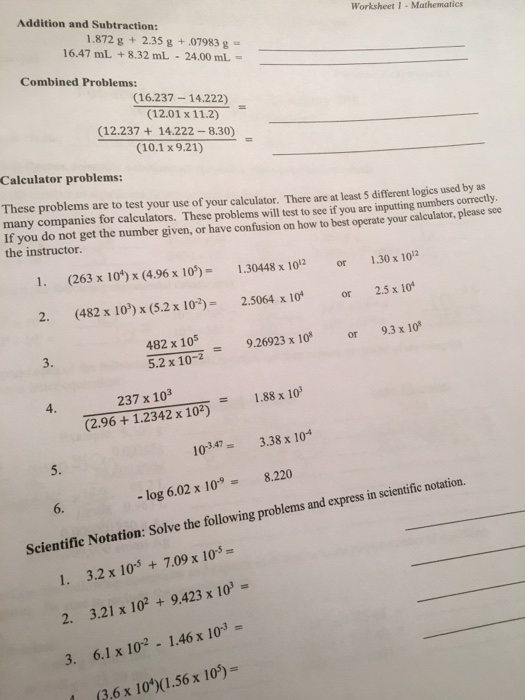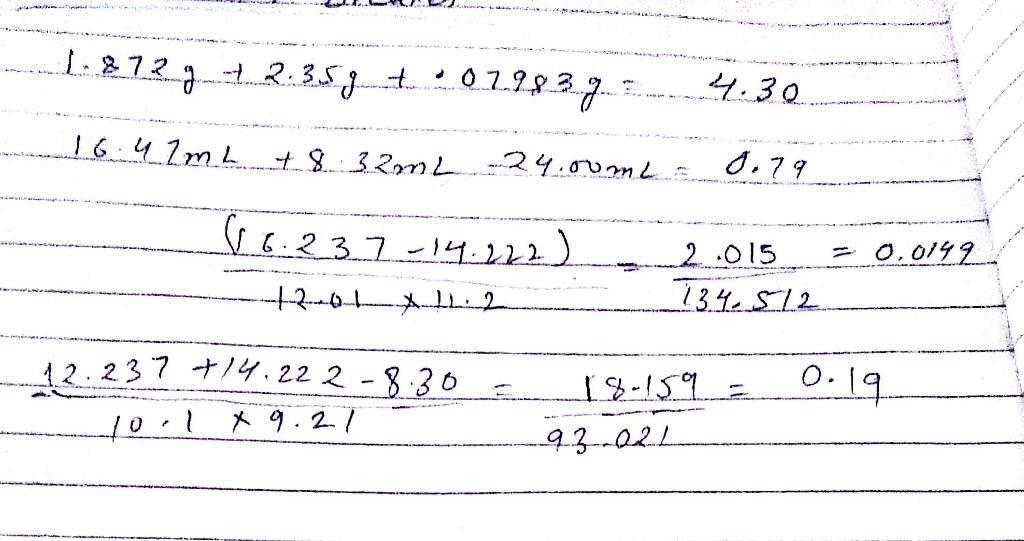# Homework Solution: These problems are to test your use of your calculator. There are least 5 different…These problems are to test your use of your calculator. There are least 5 different logics used by as many companies for calculators. These problems will test to see if you are inputting numbers correctly. If you do not get the number given, or have confusion on how to best operate your calculator, please see the instructor. (263 times 10^4) times (4.96 times 10^5) = 1.30448 times 10^12 or 1.30 times 10^12 (482 times 10^3) times (5.2 times 10^-2) = 2.5064 times 10^4 or 2.5 times 10^4 482 times 10^5/5.2 times 10^-2 = 9.26923 times 10^8 or 9.3 times 10^8 237 times 10^3/(2.96 + 1.2342 times 10^2) = 1.88 times 10^3 10^-3.47 = 3.38 times 10^-4 -log 6.02 times 10^-9 = 8.220 Solve the following problems and express in scientific notation. 3.2 times 10^-5 + 7.09 times 10^-5 = ____ 3.21 times 10^2 + 9.423 times 10^3 = _____ 6.1 times 10^-2 - 1.46 times 10^-3 = ____ (3.6 times 10^4)(1.56 times 10^5) = ____